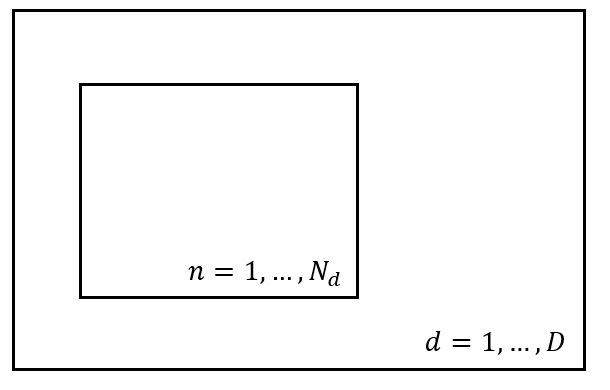# Subsampling in nested plate with varying size

Hi!

I have a model with two plates D and N. The size of the N plate depends on the value of d. Now I want to do subsampling along the N plate. Is this possible in Pyro? I cannot specify the plate size, because it depends on d.I think you can use mask to make your tensors have consistent shapes.

Could you elaborate?

For example assume I have
d = 0, N_0 = 15
d = 1, N_1 = 13
d = 2, N_2 = 16
d = 3, N_3 = 14

I could make the N plate have size 16 and use only the subsampled indices <= N_d, but then I’d have different subsample sizes.

I think you need to use a fixed subsample size. I’m not sure how to set different subsample sizes for a particular plate.

``````with plate("d", size=d):
with plate("N", size=max(N_i), subsample_size=3):
...
``````
1 Like

note also that you don’t need to use `plate`s to do subsampling. instead you can define your model without plates, handle subsampling outside of `svi.step()`, pass in subsampled data to `step()` and make sure that `sample` statements are properly weighted given the subsampling you’re doing by using poutine.handlers.scale

1 Like